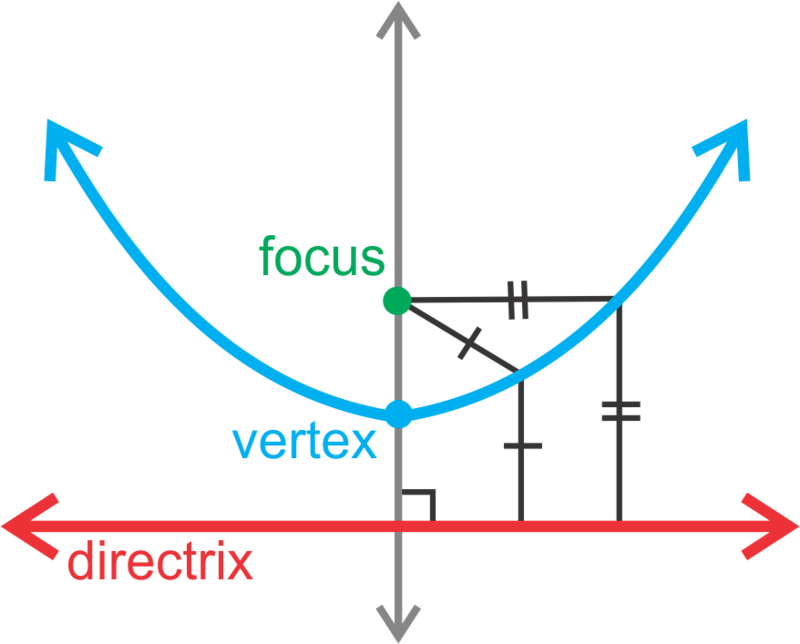# Parabolas with Vertex at the Origin

The area of a square is represented by the equation $$\ y=9 x^{2}$$. What are the focus and directrix of this equation?

# Parabolas with Vertex at the Origin

You already know that the graph of a parabola has the parent graph $$\ y=x^{2}$$, with a vertex of (0, 0) and an axis of symmetry of $$\ x = 0$$. A parabola can also be defined in a different way. It has a property such that any point on it is equidistant from another point, called the focus, and a line called the directrix.

The focus is on the axis of symmetry and the vertex is halfway between it and the directrix. The directrix is perpendicular to the axis of symmetry.Until now, we have been used to seeing the equation of a parabola like $$\ y=a x^{2}$$. In this concept, we will rewrite the equation to look like $$\ x^{2}=4 p y$$ where $$\ p$$ is used to find the focus and directrix. We will also draw the parabola with a horizontal orientation, such that the equation will be $$\ y^{2}=4 p x$$.Notice, that when the parabola opens to the left or right, the y is squared. In this concept, the vertex will be (0, 0).

Let's analyze the equation $$\ y^{2}=-12 x$$. We'll find the focus, directrix, and determine if the function opens up, down, to the left or right. Then, we'll graph the parabola.

To find the focus and directrix, we need to find $$\ p$$.  We can set $$\ -12=4 p$$ and solve for $$\ p$$.

$$\ \begin{array}{c} -12&=4 p \\ -3&=p \end{array}$$

Because $$\ y$$ is squared, we know that the parabola opens to the left or right. Because $$\ p$$ is negative, we know it is going to open to the left, towards the negative side of the x-axis. Using the pictures above, this parabola is like the second one under $$\ y^{2}=4 p x$$. Therefore, the focus is (−3, 0) and the directrix is x=3. To graph the parabola, plot the vertex, focus, directrix, and sketch the curve. Find at least one or two points on the curve to make sure your sketch is accurate. For example, because (−3, 6) is on the parabola, then (−3, −6) is also on the parabola because it is symmetrical.

Notice that the points (−3, 6) and (−3, −6) are equidistant from the focus and the directrix. They are both 6 units from each.The focus of a parabola is $$\ \left(0, \frac{1}{2}\right)$$. Let's find the equation of the parabola.

Because the $$\ p$$ value is the y-value and positive, this parabola is going to open up. So, the general equation is $$\ x^{2}=4 p y$$. Plugging in $$\ \frac{1}{2}$$ for $$\ p$$, we have $$\ x^{2}=4 \cdot \frac{1}{2} y$$ or $$\ x^{2}=2 y$$.

Now, let's find the equation of the parabola below.The equation of the directrix is $$\ y=5$$, which means that $$\ p=-5$$ and the general equation will be $$\ x^{2}=4 p y$$. Plugging in -5 for $$\ p$$, we have $$\ x^{2}=-20 y$$.

# Examples

Example 1

Earlier, you were asked to find the focus and directrix of the equation $$\ y=9 x^{2}$$.

Solution

To find the focus and directrix, we need to solve for $$\ x^{2}$$ and then find $$\ p$$.

$$\ \begin{array}{l} y&=9 x^{2} \\ \frac{1}{9} y&=x^{2} \end{array}$$

We can now set $$\ \frac{1}{9}=4 p$$ and solve for $$\ p$$.

$$\ \begin{array}{l} \frac{1}{9}=4 p \\ \frac{1}{36}=p \end{array}$$

Therefore, the focus is $$\ \left(0, \frac{1}{36}\right)$$ and the directrix is $$\ y=-\frac{1}{36}$$.

Example 2

Determine if the parabola $$\ x^{2}=-2 y$$ opens up, down, left or right.

Solution

Down; $$\ p$$ is negative and $$\ x$$ is squared.

Example 3

Find the focus and directrix of $$\ y^{2}=6 x$$. Then, graph the parabola.

Solution

Solving for $$\ p$$, we have $$\ 4 p=6 \rightarrow p=\frac{3}{2}$$. Because $$\ y$$ is squared and $$\ p$$ is positive, the parabola will open to the right. The focus is $$\ \left(\frac{3}{2}, 0\right)$$ and the directrix is $$\ x=-\frac{3}{2}$$.Example 4

Find the equation of the parabola with directrix $$\ x=-\frac{3}{8}$$.

Solution

If the directrix is negative and vertical (x=), we know that the equation is going to be $$\ y^{2}=4 p x$$ and the parabola will open to the right, making $$\ p$$ positive; $$\ p=\frac{3}{8}$$. Therefore, the equation will be $$\ y^{2}=4 \cdot \frac{3}{8} \cdot x \rightarrow y^{2}=\frac{3}{2} x$$.

# Review

Determine if the parabola opens to the left, right, up or down.

1. $$\ x^{2}=4 y$$
2. $$\ y^{2}=-\frac{1}{2} x$$
3. $$\ x^{2}=-y$$

Find the focus and directrix of the following parabolas.

1. $$\ x^{2}=-2 y$$
2. $$\ y^{2}=\frac{1}{4} x$$
3. $$\ y^{2}=-5 x$$

Graph the following parabolas. Identify the focus and directrix as well.

1. $$\ x^{2}=8 y$$
2. $$\ y^{2}=\frac{1}{2} x$$
3. $$\ x^{2}=-3 y$$

Find the equation of the parabola given that the vertex is (0, 0) and the focus or directrix.

1. focus: (4, 0)
2. directrix: x=10
3. focus: $$\ \left(0, \frac{7}{2}\right)$$
4. You have seen that earlier the basic parabolic equation was $$\ y=a x^{2}$$. Now, we write $$\ x^{2}=4 p y$$. Rewrite $$\ p$$ in terms of $$\ a$$ and determine how they affect each other.
5. Challenge Use the distance formula, $$\ d=\sqrt{\left(x_{2}-x_{1}\right)^{2}-\left(y_{2}-y_{1}\right)^{2}}$$, to prove that the point (4, 2) is on the parabola $$\ x^{2}=8 y$$.
6. Real World Application A satellite dish is a 3-dimensional parabola used to retrieve sound, TV, or other waves. Assuming the vertex is (0, 0), where would the focus have to be on a satellite dish that is 4 feet wide and 9 inches deep? You may assume the parabola has a vertical orientation (opens up).To see the Review answers, open this PDF file and look for section 10.1.

# Vocabulary

Term Definition
directrix The directrix of a parabola is the line that the parabola seems to curve away from. All points on a parabola are equidistant from the focus of the parabola and the directrix of the parabola.
focus The focus of a parabola is the point that "anchors" a parabola. Any point on the parabola is exactly the same distance from the focus as from the directrix.
Parabola A parabola is the set of points that are equidistant from a fixed point on the interior of the curve, called the '''focus''', and a line on the exterior, called the '''directrix'''. The directrix is vertical or horizontal, depending on the orientation of the parabola.